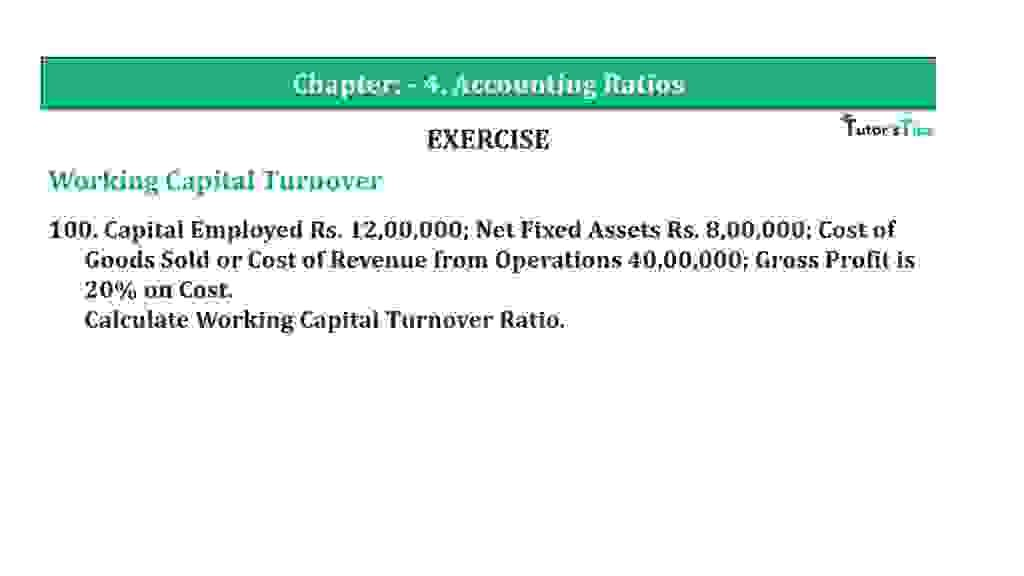# Question 100 Chapter 4 of +2-B – T.S. Grewal 12 ClassQuestion 100 Chapter 4 of +2-B

Working Capital Turnover

100. Capital Employed Rs. 12,00,000; Net Fixed Assets Rs. 8,00,000; Cost of
Goods Sold or Cost of Revenue from Operations 40,00,000; Gross Profit is 20% on Cost.
Calculate Working Capital Turnover Ratio.

### The solution of Question 100 Chapter 4 of +2-B: –

 Cost of Goods Sold = Rs. 40,00,000 Gross Profit = 20% of Cost

 Gross Profit =Rs.40,00,000 + 20 100 =Rs. 8,00,000

 Total Sales = Cost of Goods Sold + Gross Profit = Rs. 40,00,000 + Rs. 8,00,000 = Rs. 48,00,000 Working Capital = Capital Employed – Fixed Assets = Rs. 12,00,000 – Rs. 8,00,000 = Rs. 4,00,000

 Working Capital Turnover Ratio = Cost of Goods Sold Working Capital
 = Rs. 48,00,000 Rs. 4,00,000 = 12 Times

Balance Sheet: Meaning, Format & Examples

Thanks, Please Like and share with your friends

Comment if you have any question.

Also, Check out the solved question of previous Chapters: –

### T.S. Grewal’s Double Entry Book Keeping (Vol. II: Accounting for Companies)T.S. Grewal’s Analysis of Financial Statements